Beam Deflection Formula and Equations

Free Calculator To Calculate Beam Deflection

Beam Deflection Formula and Equations for Beams

Beam Deflection Equations are easy to apply and allow engineers to make simple and quick calculations for deflection. If you're unsure about what deflection actually is, click here for a deflection definition Below is a concise beam deflection table that shows how to calculate the maximum deflection in a beam. Don't want to hand calculate these? SkyCiv offers a free beam deflection calculator to help with your needs! For more powerful structural analysis software, sign up for a free SkyCiv Account and get instant access to all the free versions of our cloud structural analysis software!

Use the below beam deflection formula to calculate the max displacement in beams. Click the 'check answer' button to open up our free beam calculator. These beam displacement equations are perfect for quick hand calculations and quick designs. Find what you're looking for faster:

 Reference Max Deflection BMD Shape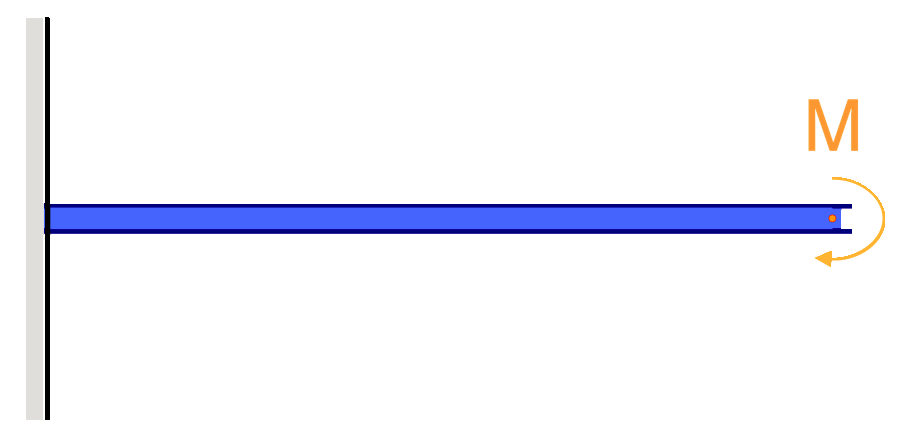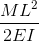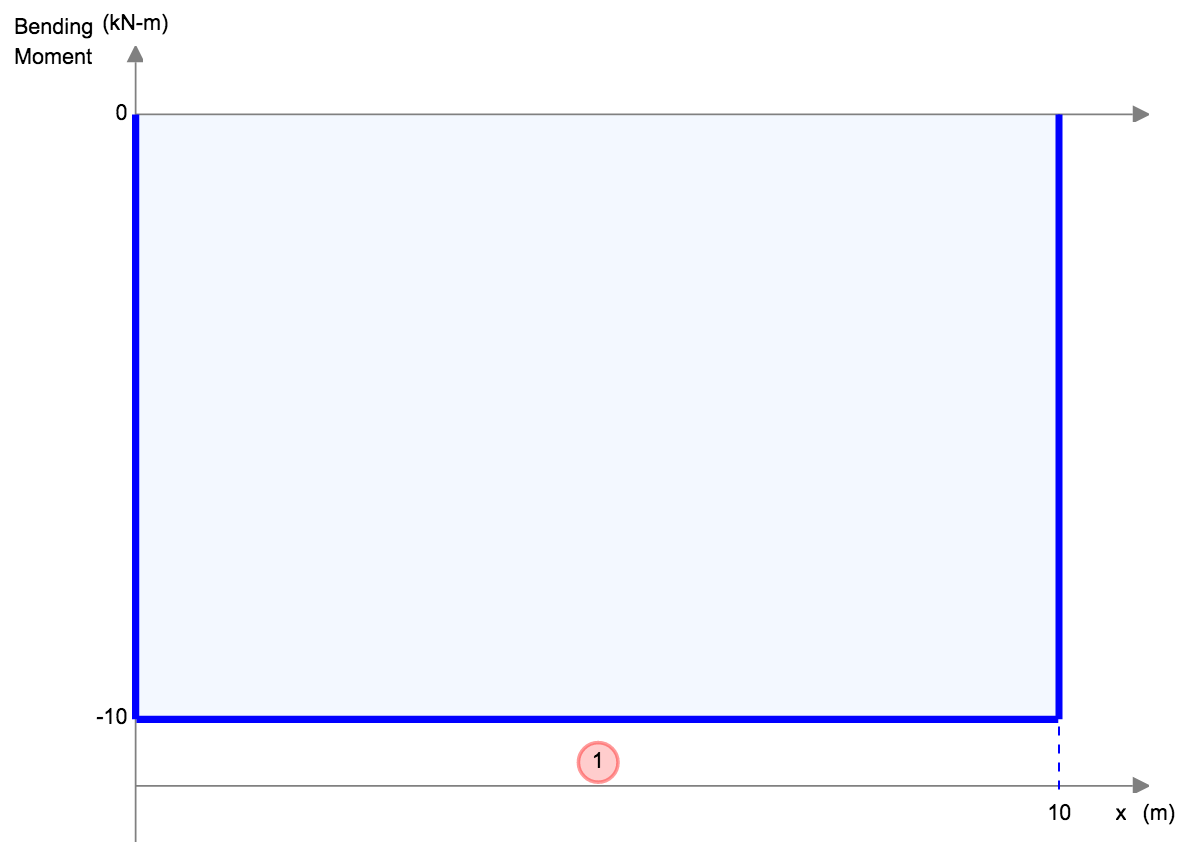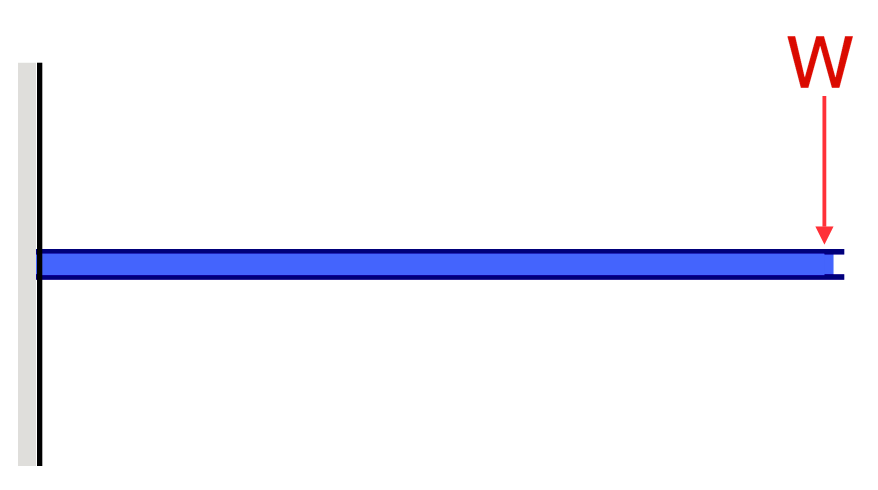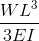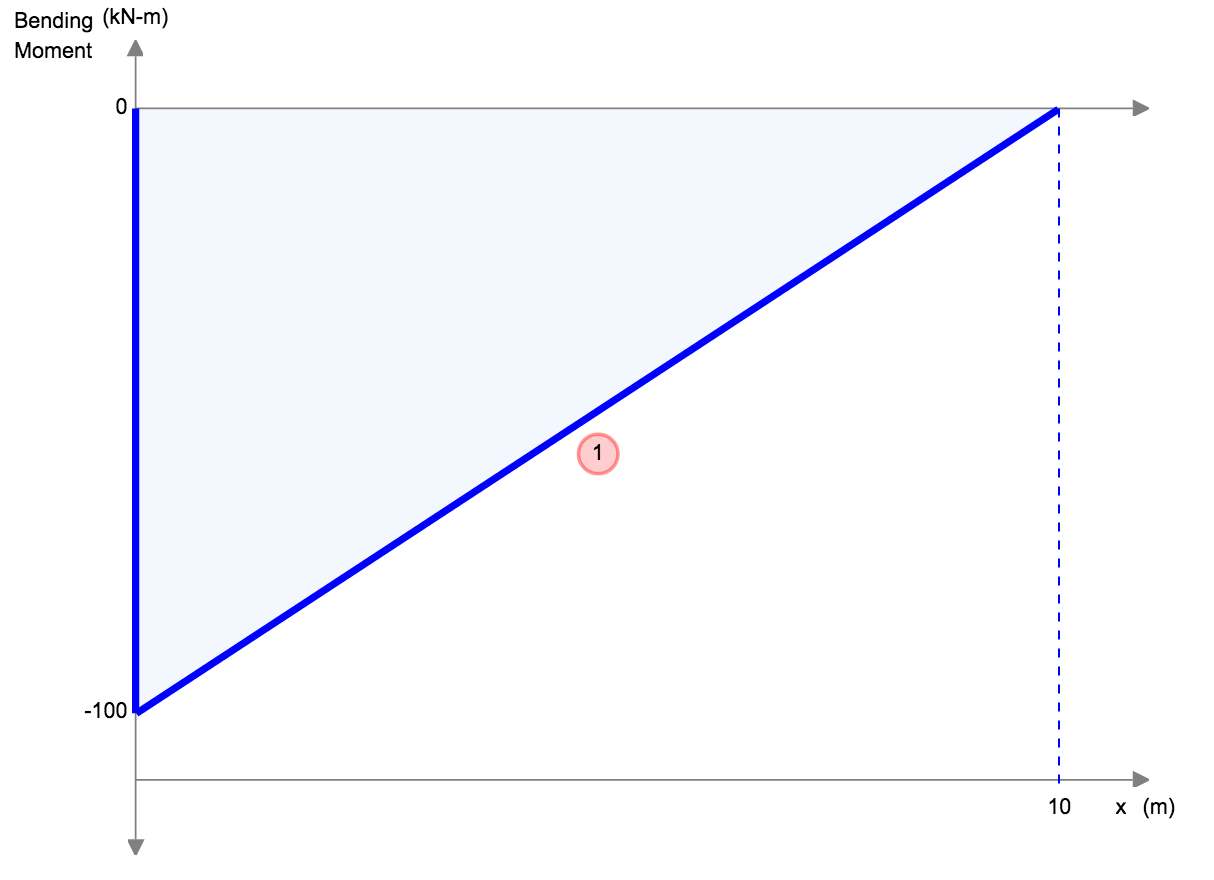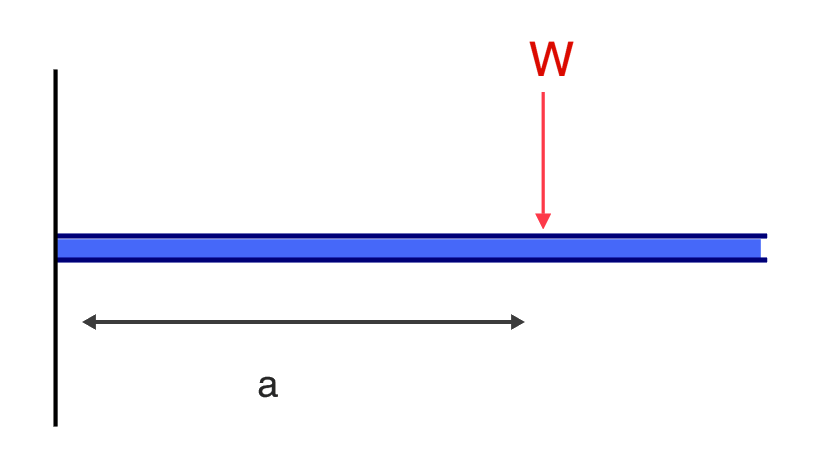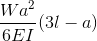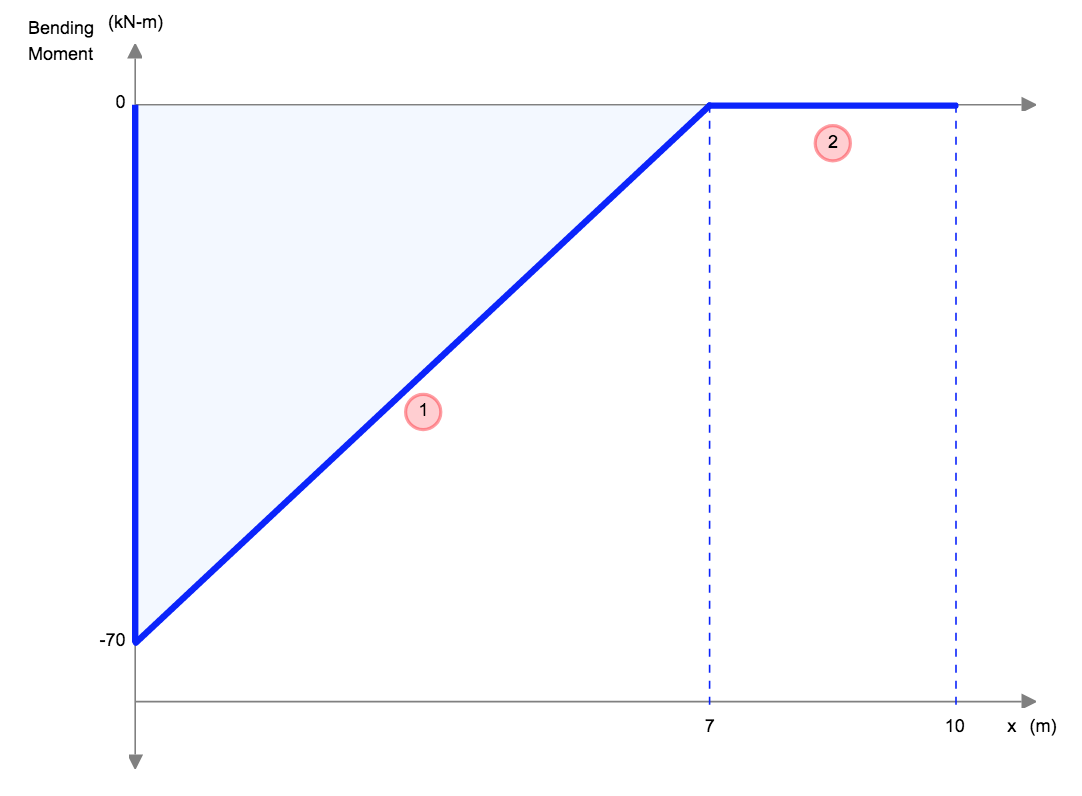Check your answers using our Free Beam Software! Cantilever Beam Deflection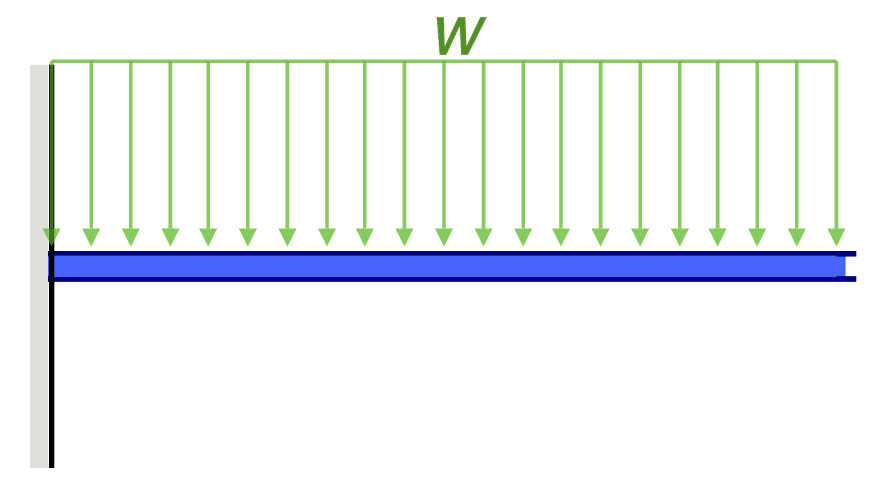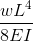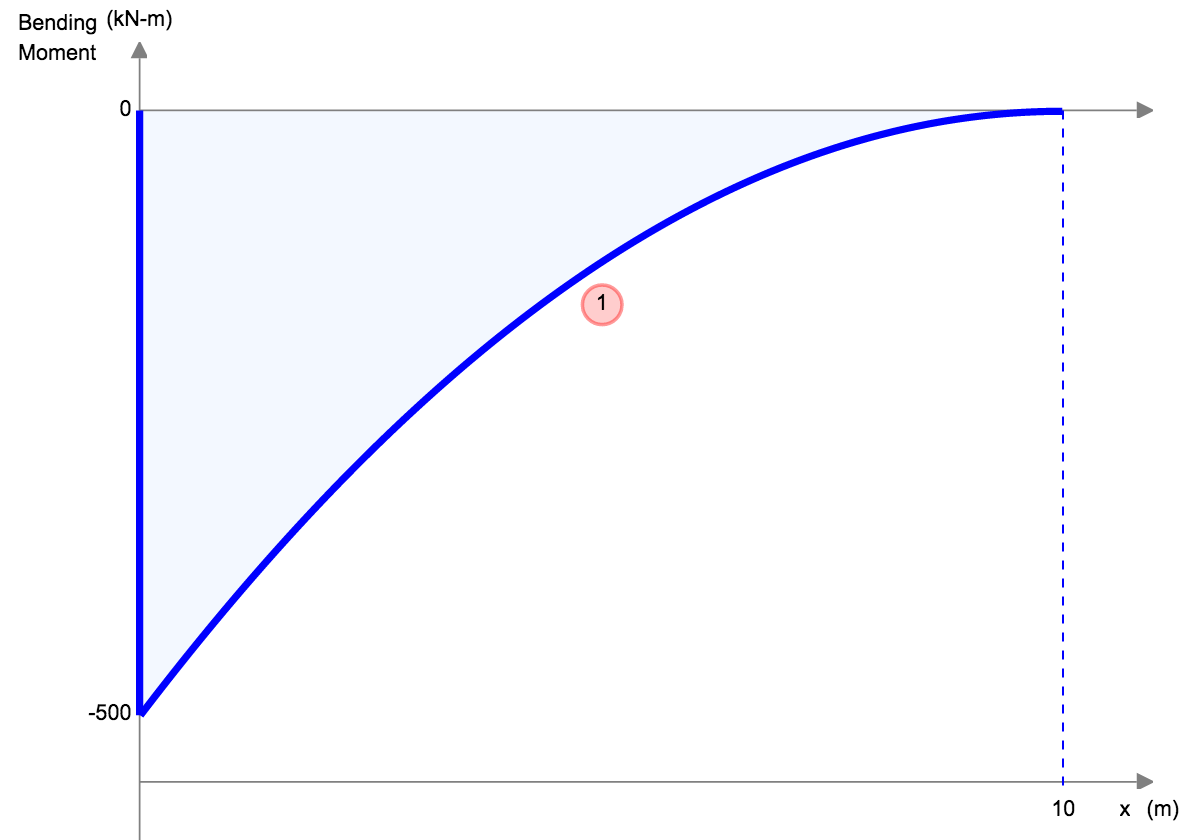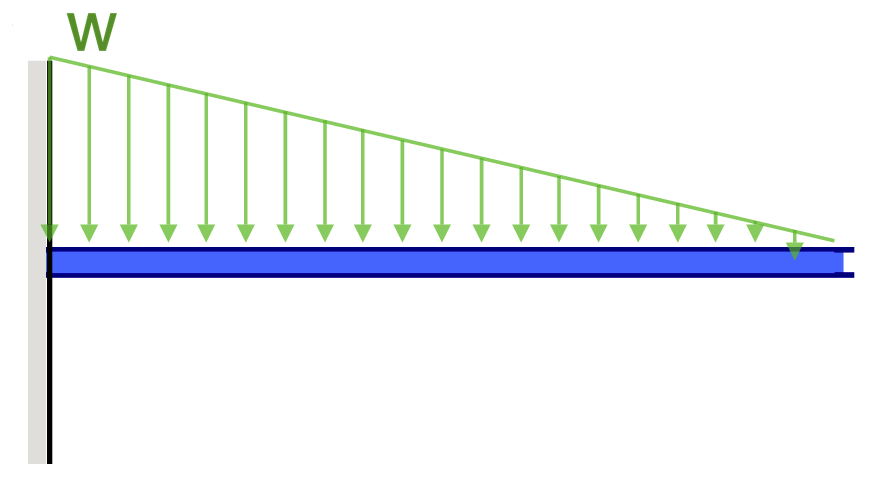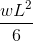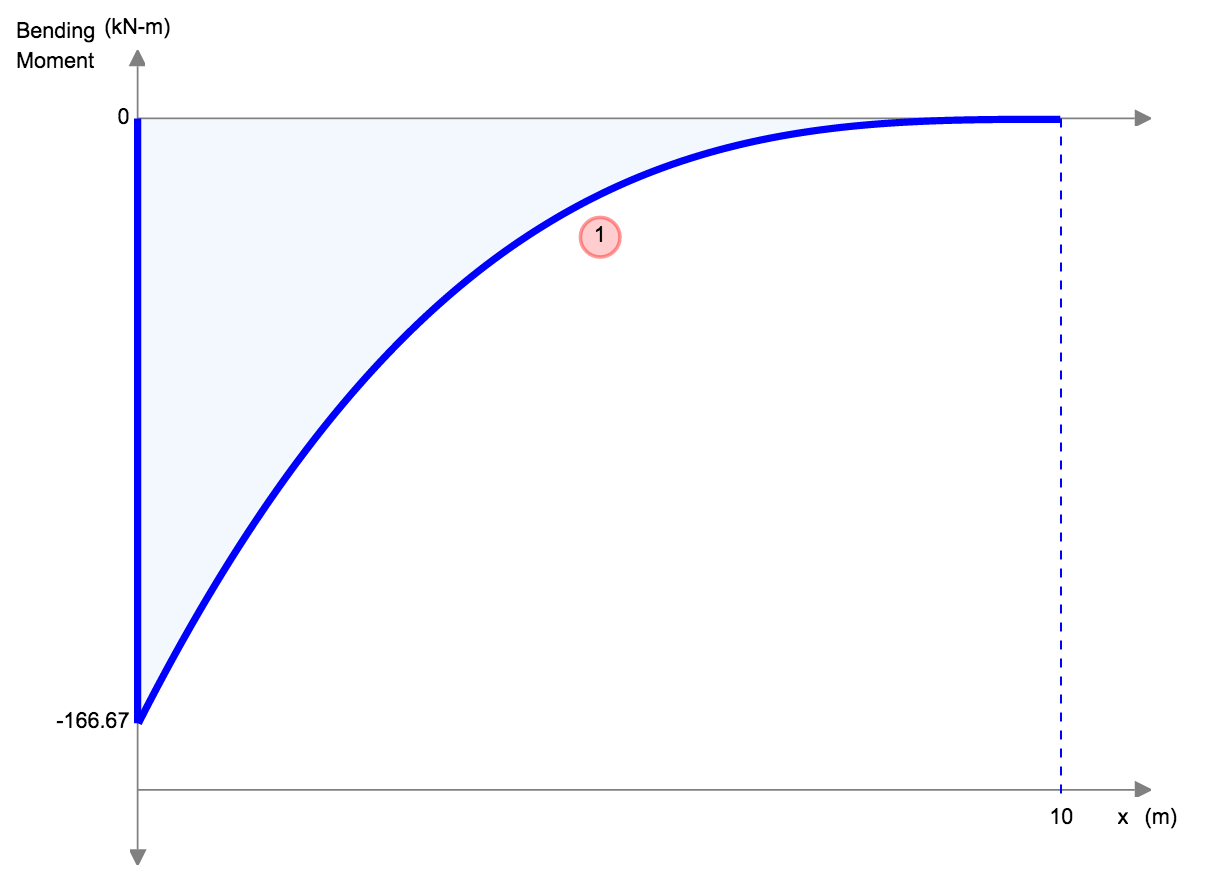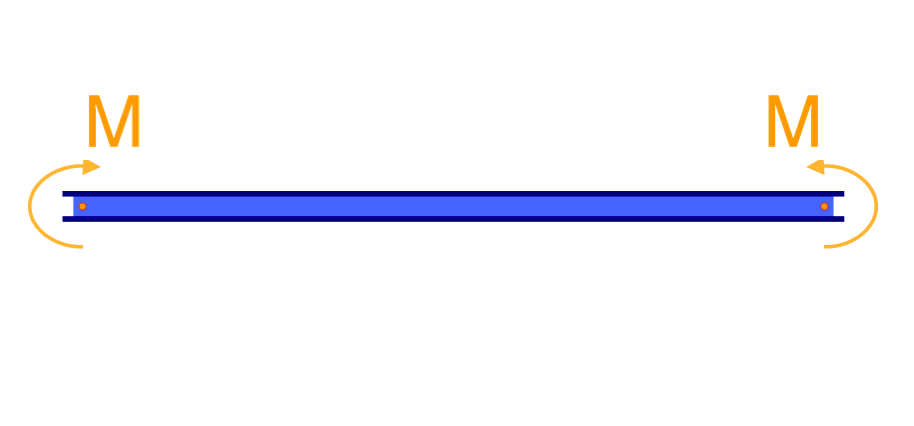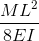- Check your answers using our Free Beam Software! Cantilever Beam Deflection Calculator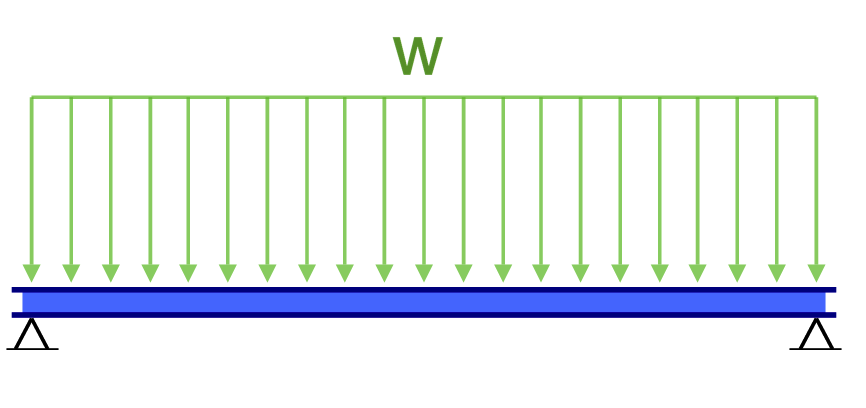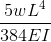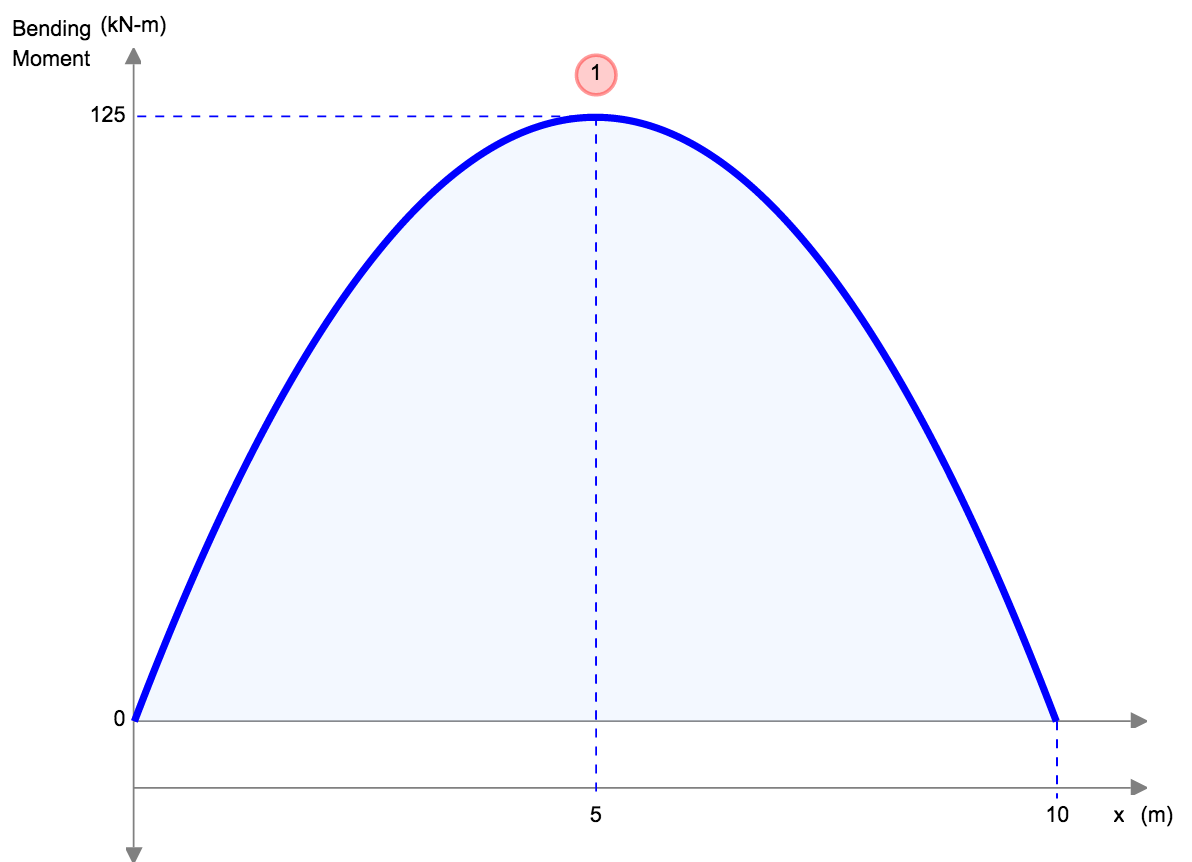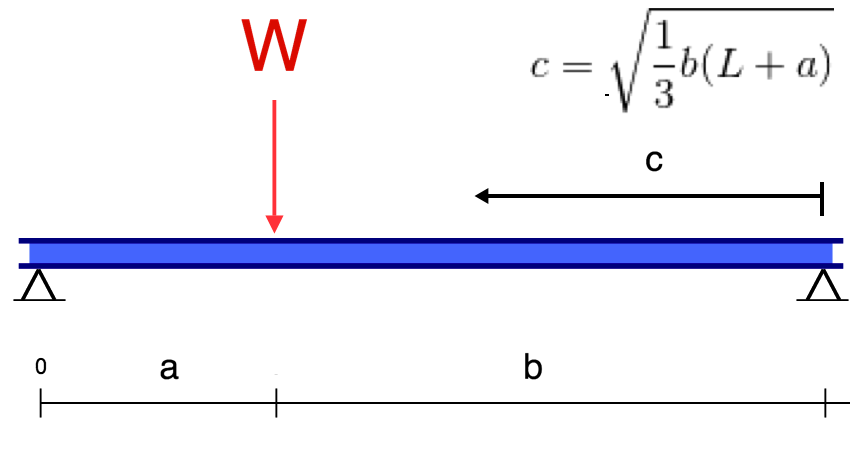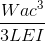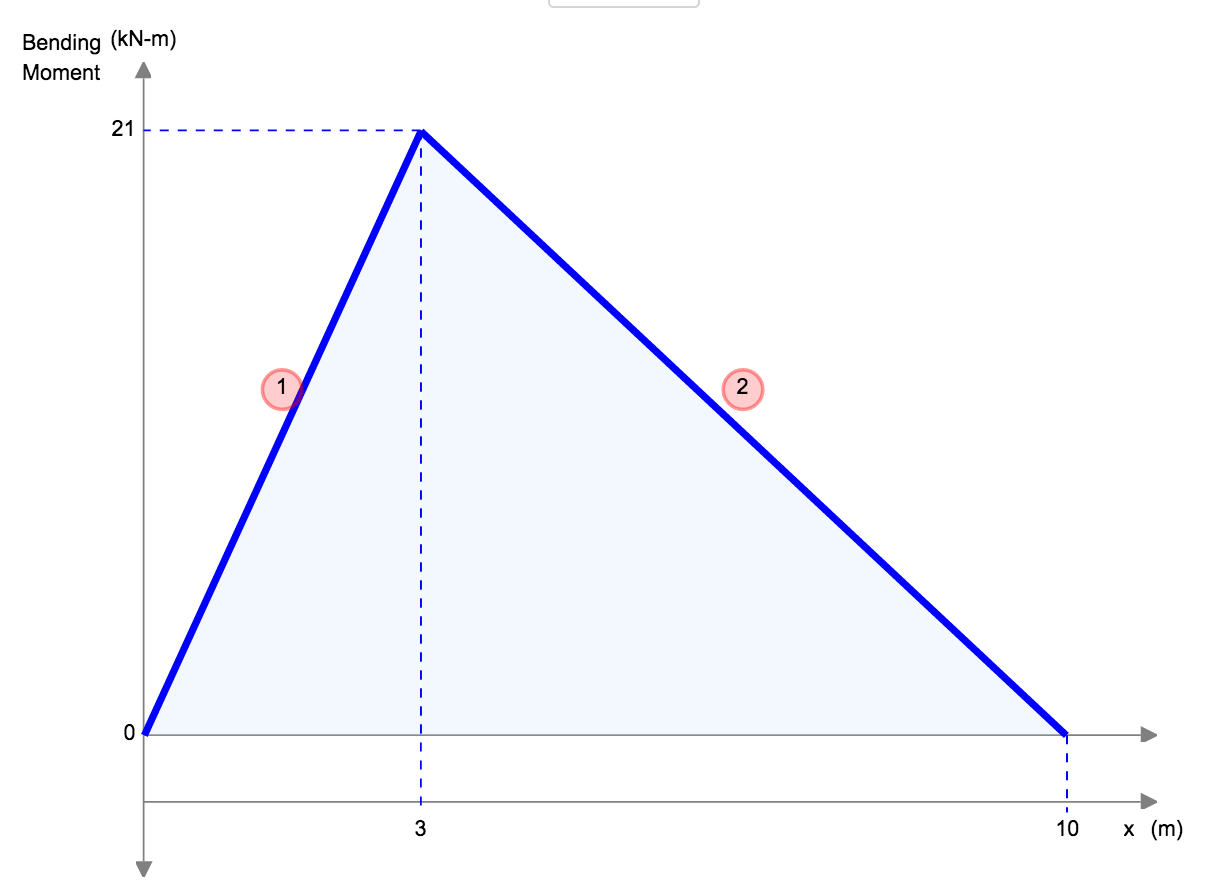Signup for Free Beam Software! Beam Deflection Calculator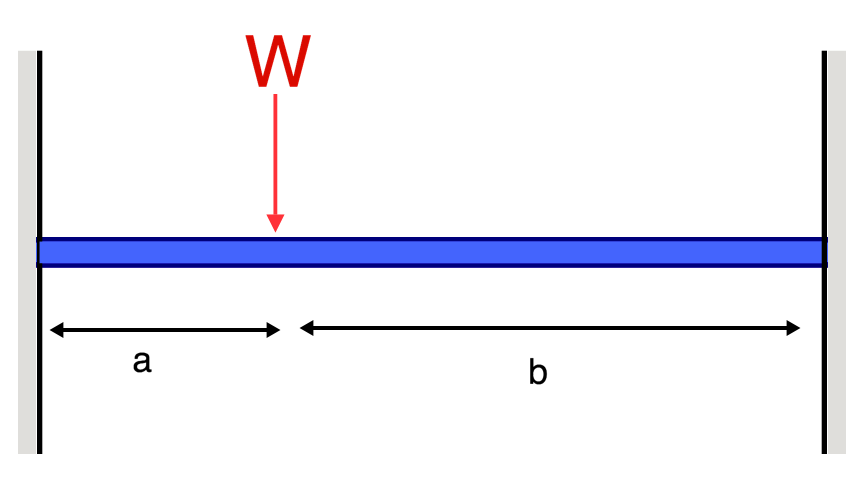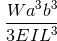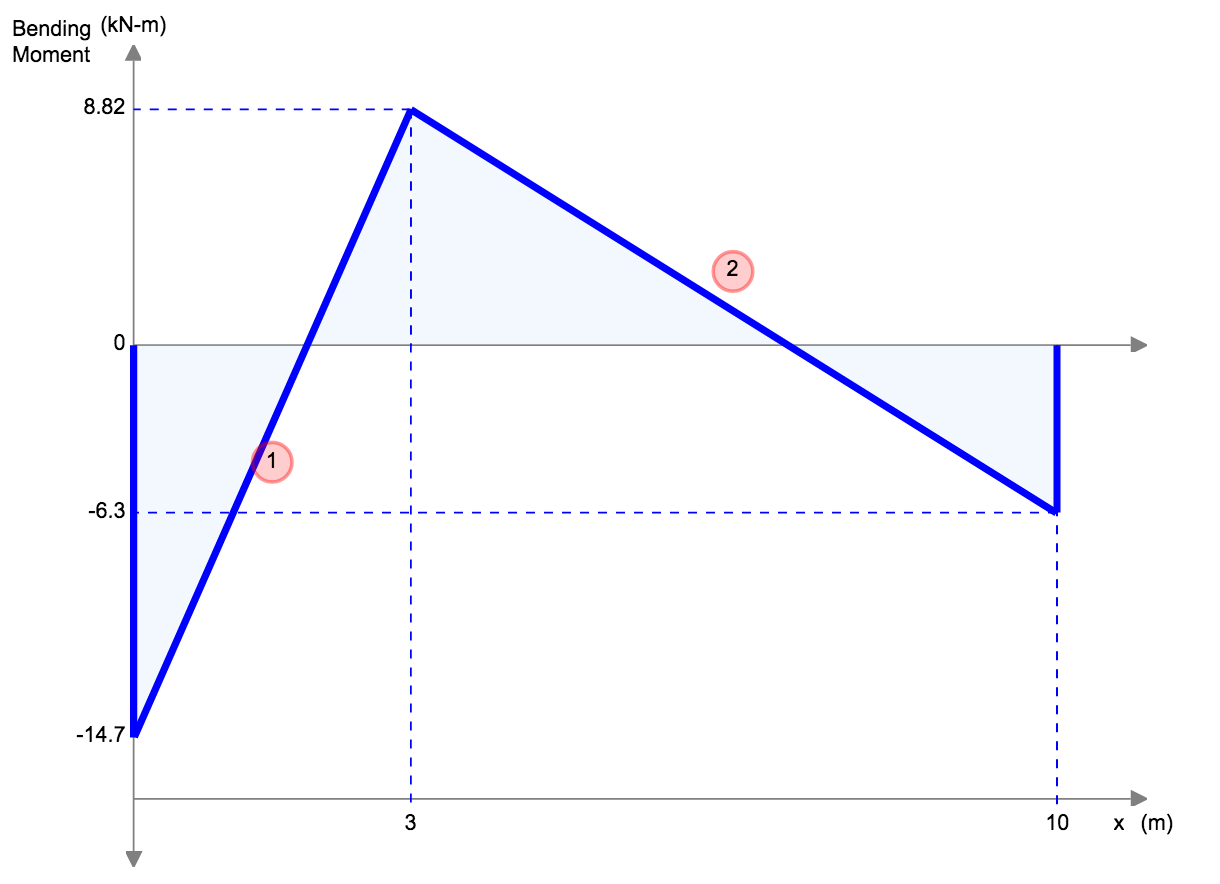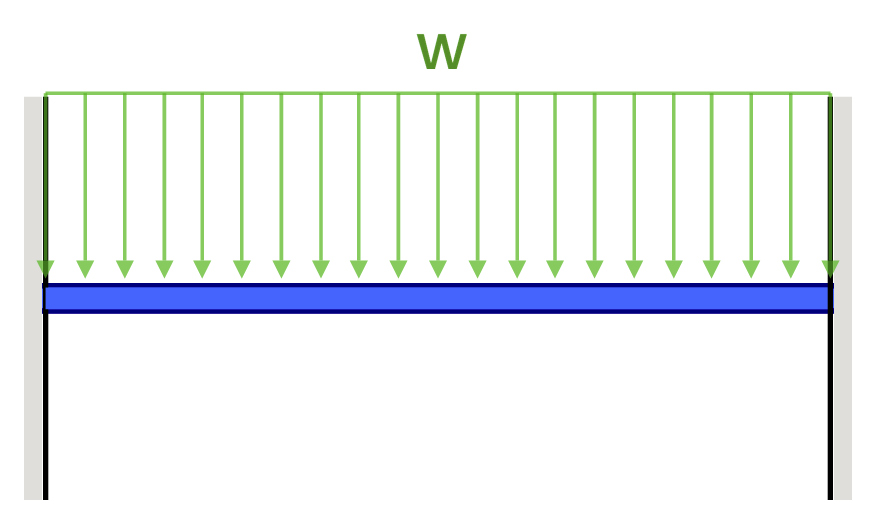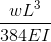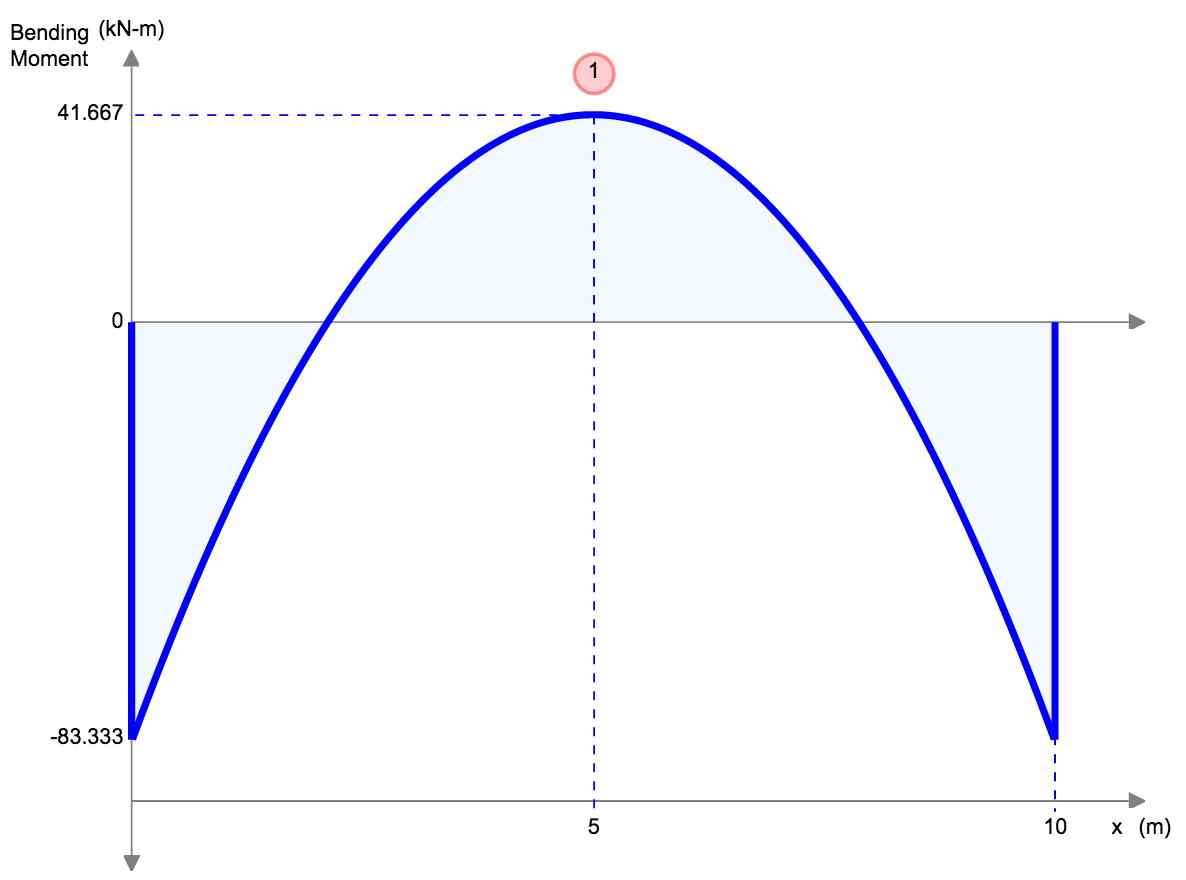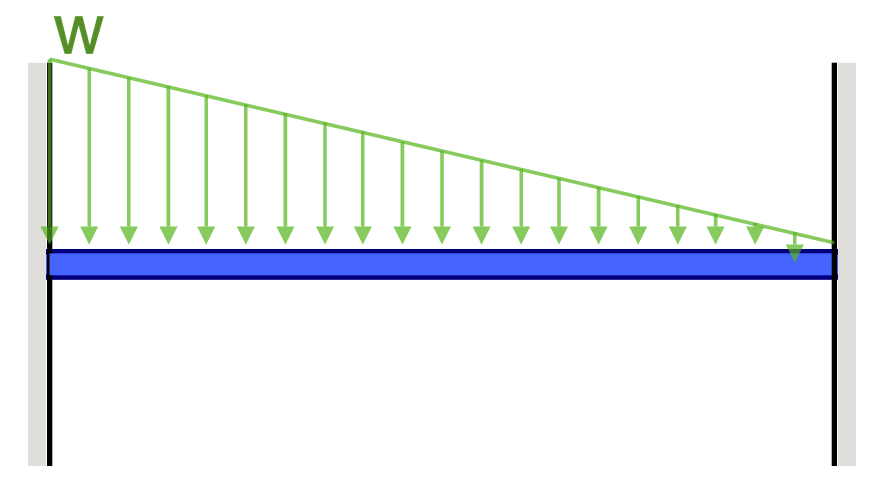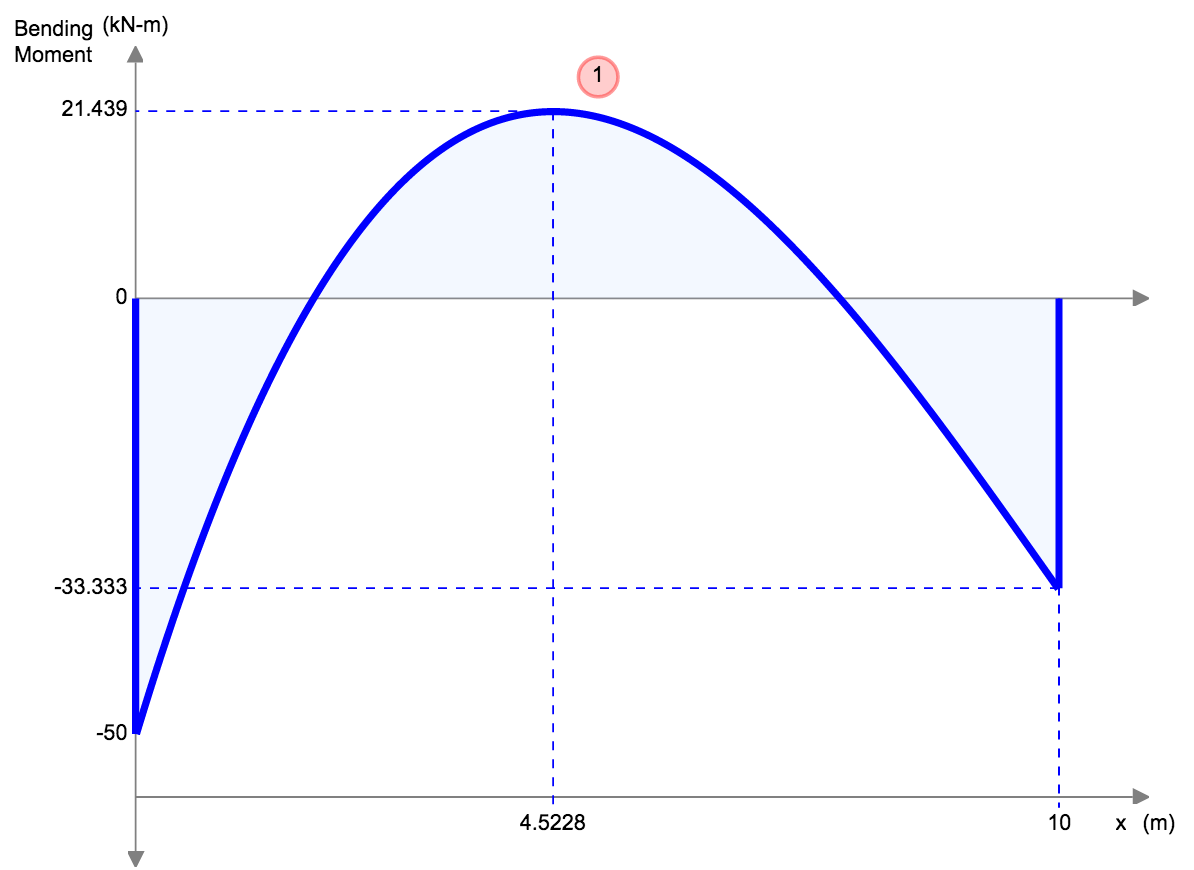Signup for Free Beam Software! Beam Deflection Calculator

As a reminder, we have a beam deflection calculator that can help you with calculating the deflection of beams. We hope you found this beam deflection table useful! Please let us know below if you'd like any more types of beam added to the list and we'll add them! We hope you find this a useful reference for you beam displacement calculations!

Free Calculator To Calculate Beam Deflection